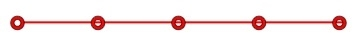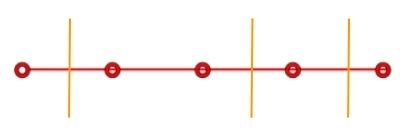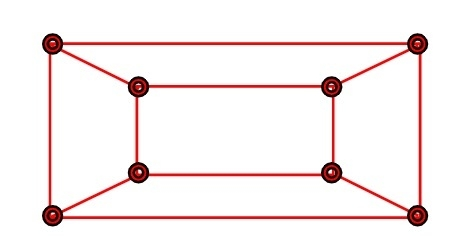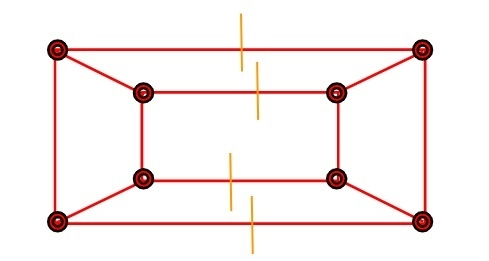# C++ program to Calculate the Edge Cover of a Graph

Given a n number of vertices of a graph, the task is to calculate the edge cover of the graph. Edge cover is to find the minimum number of edges required to cover every vertex of the graph.

Like we have n = 5

Then its graph will be like −So its edge cover is 3Let’s take another example where the n is 8And its edge cover will be:4## Example

Input: n= 5
Output: 3
Input: n= 8
Output: 4

Approach used below is as follows

• Take the input from the user
• Find the ceiling value of the result of number of vertices by dividing it by 2.0
• Return and print the result.

## Algorithm

Start
Step 1-> declare function to calculate the edge cover of a graph
int edge(int n)
set float val = 0
set val = ceil(n / 2.0)
return val
step 2-> In main()
set int n = 10
call edge(n)
Stop

## Example

Live Demo

#include <bits/stdc++.h>
using namespace std;
// Function to calculates Edge Cover
int edge(int n) {
float val = 0;
val = ceil(n / 2.0);
return val;
}
int main() {
int n = 10;
cout<<"minium number of edges required are :"<<edge(n);
return 0;
}

## Output

IF WE RUN THE ABOVE CODE IT WILL GENERATE FOLLOWING OUTPUT

minium number of edges required are :5